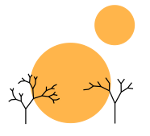# Not Everything is a Function in Haskell

Debian Logo: Software in the Public Interest, Inc. "The Debian Open Use Logo." 1999. Under a GNU Lesser General Public License (NOT a CC-BY 3.0 License).

Screenshot: cmus Project. "Untitled." 2013. Under a GNU General Public License v3.0 (NOT a CC-BY 3.0 License).

Logo + Screenshot: Shuang, Rimu. "Untitled." Mar 19, 2014. Under a GNU General Public License v3.0 (NOT a CC-BY 3.0 License).

### moving, django, hakyll

A common misconception in Haskell is that everything is a function (a nice example of this is seydar on Reddit asking “Would it be heresy to henceforth call ‘y’ a function with no arguments…” here. I’m still learning Haskell, and I found at the very beginning that this was a nice way of thinking about things in Haskell. After all it tied together in a very nice way. All of a sudden it made perfect sense to pass functions to other functions because it was the exact same thing as passing non-function arguments. The syntax of Haskell also started making a ton of sense. If f x y = z has type a -> b -> c and f x = z has type a -> c, then naturally f = z has type c. So then if we have something such as (+) 3 2 which has type Int -> Int -> Int and 3 just has type Int, then maybe it’s just a nullary function!

Aha, they’re all the same thing; they’re all functions! But they’re not. Conal Elliott has a nice article about why this isn’t the case and goes into the motivations that he thinks are behind such thinking.

I realized today, as I progress further along the road of learning Haskell, that the monadic behavior behind what we usually write using do notation in Haskell, provides nice justification for why not everything in Haskell is a function. In particular, if, for example 3 was a function, then in Haskell, we would represent it as () -> Int, to describe the fact that it took no arguments. So what’s wrong with that? Maybe it gunks up our type system a little to say that Int and () -> Int can both be used to describe the same thing (even though they themselves might be distinct from one another), but Haskell’s already confusing enough right?

Well let’s take a look at the following short function

counter :: Int -> IO ()
counter x = putStrLn (show x) >>= (\y -> counter (x + 1))

Equivalently we might write

counter :: Int -> IO ()
counter x = do
putStrLn (show x)
counter (x + 1)

This function takes a number and then counts up forever, printing the result of each computation to the screen, which would yield something like the following if started at 1.

$1$ 2
$3$ 4
\$ 5
...

Note that (\y -> counter (x + 1)) has type () -> IO (). If we were to state that () -> a and a can refer to the same things, then this would mean that in fact (\y -> counter (x + 1)) could also be said to have type IO (). Yet counter (x + 1) has type IO () and it is most emphatically not (\y -> counter (x + 1)). In fact you’ll get a type error, and for good reason, because from the perspective of monads, it makes no sense to bind a result of IO () to something of type IO ().

Hence although it might be nice mathematically for everything in Haskell to be a function (and in fact it seems there are mathematical frameworks in which this is possible), this is not the case in real life.